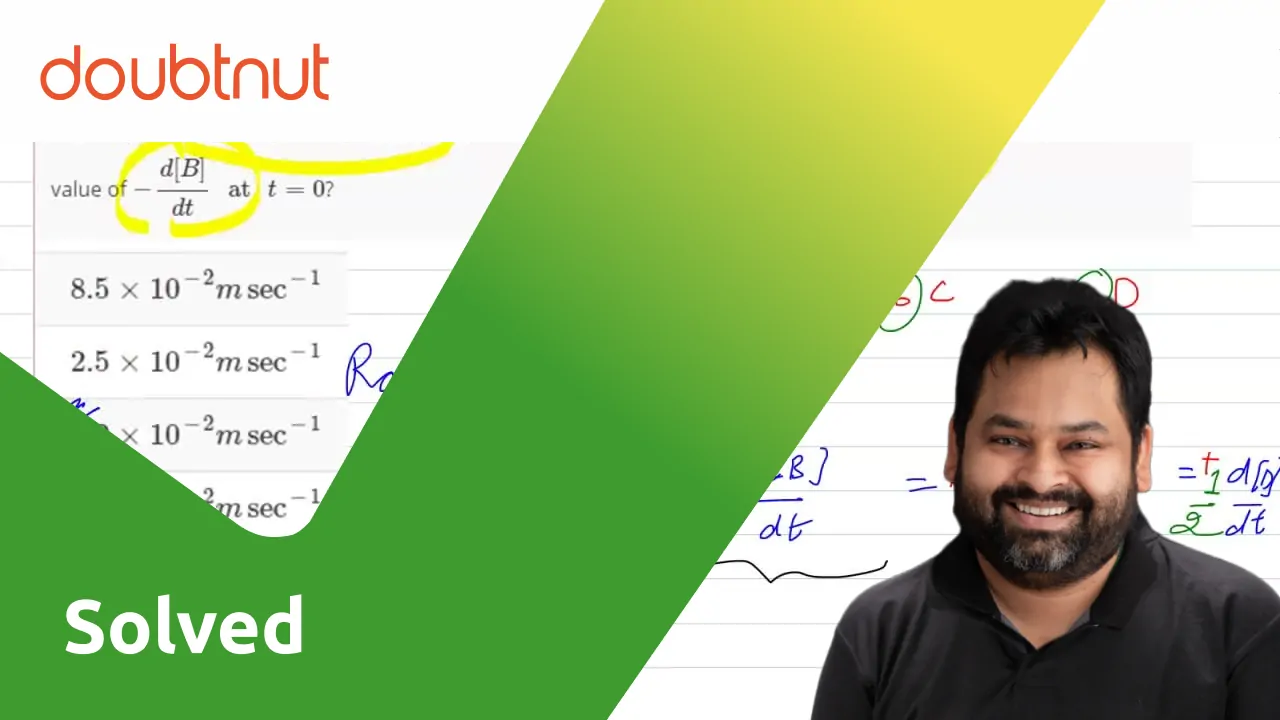Doubtnut App - Get 50 Lakh+ video solutions better than GoogleInstall Now

# In the reaction, A+2B→6C+2D, if the initial rate −d[A]dt at t = 0 is 2.6×10−2msec−1, what will be the value of −d[B]dt at t=0?

A

8.5×102msec1

B

2.5×102msec1

C

5.2×102msec1

D

7.5×102msec1

Text Solution
Verified by Experts

## From the reaction it is evident that when a mole of A is reacting, 2 moles of B must react. Hence the decrease in the concentration of B must be twice that of A ∴−d[B]dt=2[−d[A]dt]=2×10−2=5.2×10−2sec−1